Recursive Function Theory

# Recursive Function Theory Notes | Study Theory of Computation - Computer Science Engineering (CSE)

## Document Description: Recursive Function Theory for Computer Science Engineering (CSE) 2022 is part of Theory of Computation preparation. The notes and questions for Recursive Function Theory have been prepared according to the Computer Science Engineering (CSE) exam syllabus. Information about Recursive Function Theory covers topics like and Recursive Function Theory Example, for Computer Science Engineering (CSE) 2022 Exam. Find important definitions, questions, notes, meanings, examples, exercises and tests below for Recursive Function Theory.

Introduction of Recursive Function Theory in English is available as part of our Theory of Computation for Computer Science Engineering (CSE) & Recursive Function Theory in Hindi for Theory of Computation course. Download more important topics related with notes, lectures and mock test series for Computer Science Engineering (CSE) Exam by signing up for free. Computer Science Engineering (CSE): Recursive Function Theory Notes | Study Theory of Computation - Computer Science Engineering (CSE)
 1 Crore+ students have signed up on EduRev. Have you?

Recursive Function Theory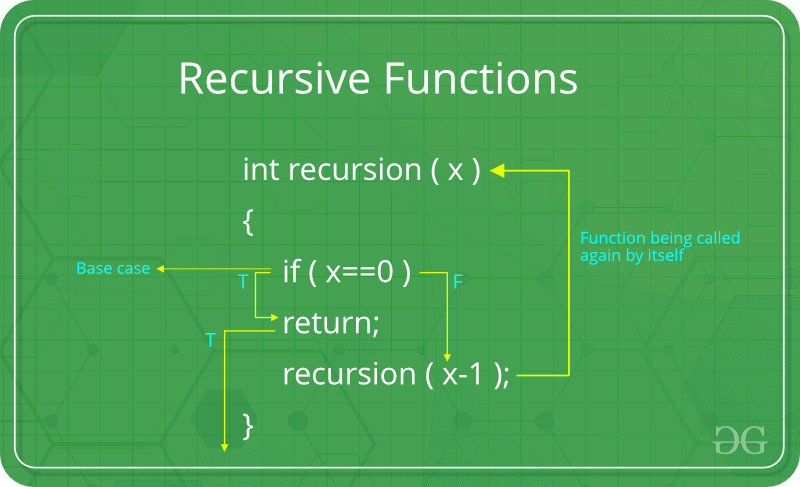A function that calls itself directly or indirectly is called a recursive function. The recursive factorial function uses more memory than its non-recursive counter part. Recursive function requires stack support to save the recursive function calls.

• Recursion leads to compact
• It is simple
• It is easy to understand
• It is easy to prove correct

Functions
The concept of a function is a fundamantal topic in mathematics.
A function or a total function f : X →Y is a rule that assigns to all the elements of one set, X, a unique element of another set, Y.
The first set, X is called the domain of the function, and the second set, Y is called its range.
For example, f(x) = 2x + 1 is a function defined on one variable, x. Imagine we say that f is over all natural numbers only. Then the following diagram shows the function: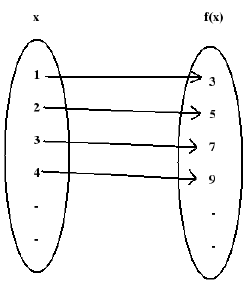Here the domain of f is {1, 2, 3, 4, ......}
Range of f is {3, 5, 7, 9, ........}.
f(x; y) = x+ 2y is a function on 2 variables, x and y.

f(x, y, z) = x + 2y3 + 7z is a function on 3 variables, x, y and z.

Total Function
The above three examples were total functions. A total function from X to Y is a rule that assigns to every element of X, a unique element of Y.
f : X → Y
For example, f(x) = 2x3
In all our discussions, we assume that f is over all natural numbers, then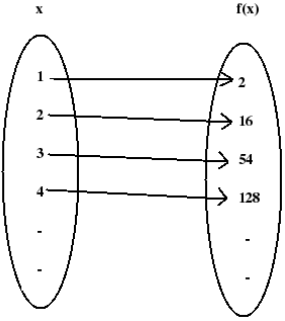Here the domain of f is {1, 2, 3, 4 .....}.
That is, the domain contains all the elements of x. So f is a total function.

Partial Function
Here, to extend the class of computable functions, we use partial functions.
A partial function, f : X→ Y

is a rule that assigns to some elements of X, a unique element of Y. For other elements, there may not be any corresponding element in Y.
Example 1: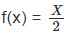Suppose f is defined over natural numbers. Then f is a partial function. this can be seen from the following diagram.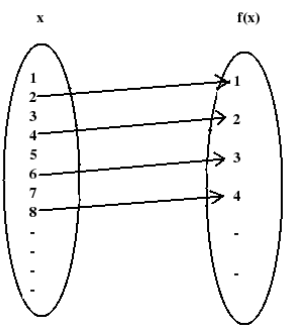In the above diagram, domain of f is {2, 4, 6, 8, ....}
Here the domain is not equal to the set X. So f is a partial function.

Example 2:
f(x, y) = x − y
f is defined over N. f is a partial function.

This is because when x=6, y=8, f(x,y) = -2
-2 is not a natural number. So f is not defined for x=6, y=8. f is a partial function.

Recursive Functions
Consider the example,
f(n) = n2 + 1
Here, given any value for n, multiply that value by itself and then add one. Here function is defined in a recursive way. Value of f can be computed in a mechanical fashion.

Example 1:
Factorial of a number is defined as,
n! = n (n-1) (n-2) . . . . . . 1.
This is an explicit definition. Another way to define factorial is,
0! = 1
n! = n (n-1)!
for n∈N, This definition is recursive because to find the factorial at an argument n, we need to find the factorial at some simpler argument (n-1).

Example 2:
Exponentiation can be defined as, x
n = x.x.x.x......x (n times)
This exponentiation function can be recursively defined as,
x0 = 1
xn = x.xn−1
for n∈N. This is a recursive definition for exponentiation.

In all our discussions, a function, f is defined over natural numbers (N) only.

Primitive Recursive Functions
A function, f is called a primitive recursive function,
i) If it is one of the three basic functions, or,
ii) If it can be obtained by applying operations such as composition and recursion to the set of basic functions.

The basic functions and operations are explained below:

1. Basic Functions

• Zero Function
Z(x) = 0 is called Zero function.
Example: We may write, Z(8) = 0
• Successor Function
The successor function is S(x), defined as
S(x) = x+1
Thus the value of S(x) is the integer next in sequence to x.
For example,
S(4) = 5, S(29) = 30
• Projector Function
Projector function, Pk is defined as,
Pk(x1, x2, ..........xk, ...........xn) = xk
For example,
P3(8, 5, 6, 4) = 6
P6(6, 34, 7, 2, 45, 23, 22) = 23

2. Operations
We can build complicated functions from the above basic functions by performing operations such as, composition, and recursion.

• Composition
If functions, f1, fand g are given, then the composition of g with f1, f2 is given by,

h = g(f1, f2).

In general, If functions f1, f2 .....fand g are given, then the composition of g with f1, f2 .....fk is h = g(f1, f2 , f3......fk)

Example 1:
Let  g(n) = n2,  h(n) = n + 3
Find the composition of h with g.
The composition of h with g is,
f(n) = h[g(n)]
= h[n2]
= n2 + 3

Example 2:
Let g(n) = n2,  h(n) = n + 3
Find the composition of g with h.
f(n) = g[h(n)]
= g[n + 3]
= (n + 3)2

Example 3:
Let, f1(x, y) = x + y
f2(x, y) = 2x
f3(x, y) = xy, and
g(x, y, z) = x + y + z
be functions over N.
Find the composition of g with f1, f2 , f3.
h(x, y) = g(f1, f2 , f3)
= g (x + y, 2x, xy)
= x + y + 2x + xy
= 3x + y + xy

• Recursion
A function f can be constructed using recursion from the functions g and h as,
f(x, 0) = g(x)
f(x, y + 1) = h [x, y, f(x, y)]
In the above, f is of two variables. g is of one variable and h is of 3 variables.

In general, a function of n+1 variables is defined by recursion if there exists a function g of n variables and a function h of n+2 variables.
f is defined as,
f(x1, x2, .....xn, 0) = g(x1, x2, ...xn)
f(x1, x2, .....xn, y + 1) = h [x1, x2, ..........xn, y, f(x1, x2, ..........xn, y) ]

Example 1:
Addition of integers can be defined using recursion as,
add(x, 0) = x
add(x, y + 1) = add(x, y) + 1

Example 2:
The function multiplication can be defined using recursion operation as,
prod(x, 0) = 0
prod(x, y + 1) = add [x, prod(x, y) ]
Above examples show how recursion operation is applied to a set of functions.

Primitive Recursive FunctionsNow we learned basic functions such as zero function, successor function and projector function, and operations such as composition and recursion.
Again, a function, f is a primitive recursive function if either,
i. it is one of the basic functions, or
ii. it is produced by performing operations such as composition and recursion to the basic functions.

Example 1:
The addition function, add(x,y) is a primitive recursive function because,
add(x, 0) = x
= P1(x)
add(x, y + 1) = add(x, y) + 1
= S[P3(x, y, add(x, y))]

Thus add(x, y) is a function produced by applying composition and recursion to basic functions, Pk and S.

Example 2:
The multiplication function mult(x, y) is a primitive recursive function because,
mult(x, 0) = 0
= Z(x)
mult(x, y + 1) = add [x, mult(x, y) ]
= add[P1(x, y, mult(x, y)), P3(x, y, mult(x, y))]
From the previous example, add() is a primitve recursive function.
From the above, mult(x,y) is produced by performing operations on basic functions and add() function.
So mult(x,y) is a primitive recursive function.

Example 3:
The factorial function,
f(n) = n!
is a primitive recursive function. This can be proved using mathematical induction.
Basic Step:
For n = 0,
f(0) = 0! = 1
= P1(1)
Induction Hypothesis:
Assume that f(p) is a primitive recursive function.
Induction Step:
f(p+1) = (p+1)!
= p! (p+1)
= f(p) (p+1)
= mult [ f(p), p+1 ]
= mult [ f(p), S(p) ]
In the above, f(p) is primitive recursive from induction hypothesis.
S(p) is primitive recursive, since it is the successor function. mult() is primitve recursive from the previous example.
So we can conclude that
f(n) = n!
is primitive recursive.

Example 4:
Show that function f1(x, y) = x + y is primitive recursive.
f1(x, 0) = x + 0
= x
= P1(x)
f1(x, y + 1) = x + (y + 1)
= (x + y) + 1
= f1(x, y) + 1
= S[f1(x, y)]
= S[P3(x, y, f1(x, y))]

Since f1(x, 0) and f1(x, y + 1) are primitive recursive, f1(x, y) = x + y is primitive recursive.

Example 5:
Show that the function, f1(x, y) = x ∗ y is primitive recursive.
f2(x, 0) = x ∗ 0
= 0
= Z(x)
f1(x, y + 1) = x ∗ (y + 1)
= (x ∗ y) + x
= f2(x, y) + x
= f1[f2(x, y), x] from Example 4.
= f1[P3(x, y, f2(x, y)), P1(x, y, f2(x, y))]

Since f2(x, 0) and f2(x, y + 1) are primitive recursive, f2(x, y) = x ∗ y is primitive recursive.

Example 6:
Show that the function, f(x, y) = xis a primitive recursive function.

f(x, 0) = x0
= 1
= P1(1)
f(x, y + 1) = xy+1
= xy.x1
= x.xy
= x.f(x, y)
= P1(x, y, f(x, y)) ∗ P3(x, y, f(x, y))
= mult [P1(x, y, f(x, y)), P3(x, y, f(x, y)) ]
mult() is a primitive recursive function as we found earlier.
Since f(x, 0) and f(x, y + 1) are primitive recursive, f(x, y) = xis primitive recursive.

Partial Recursive Functions
A function, f is a partial recursive function if either,
i. it is one of the basic functions, or
ii. it is produced by performing operations such as composition, recursion and minimization to the basic functions.

• Basic Functions: We learned three basic functions such as,
- Zero function, Z(x)
- Successor function, S(x)
- Projector function, Pk
• Operations
We learned the operations composition and recursion earlier. A new operation now comes, minimization. Minimization μ is the minimization operator.

Let a function g(x,y) is defined over two variables, x and y.
Then minimization operator, μ is defined over g (x, y) is as follows:
μy[g(x, y)] =smallest y such that g (x, y) = 0.

Using the minimization operation, a function f(x) can be defined for some y;
we can write, f(x) = μy[g(x, y)]

Example 1:
Let g(x,y) is given in the following table:
g(0,0)=5   g(1,0)=5   g(2,0)=8                 g(3,0)=1
g(0,1)=4   g(1,1)=6   g(2,1)=5                 g(3,1)=2
g(0,2)=6   g(1,2)=0   g(2,2)=undefined   g(3,2)=0
g(0,3)=0   g(1,3)=3   g(2,3)=0                 g(3,3)=4
g(0,4)=1   g(1,4)=0   g(2,4)=7               g(3,4)=undefined
Then,
f(0) = 3,
[ because g(0,3) = 0]
Minimization operation is denoted by μ[g(0, 3) = 0] = 3
f(1) = 2
[ 2 is the minimum y that g(1,2)=0; Though g(1,4)=0 also; ]
Minimization operation is denoted by μ[g(1, 2) = 0] = 2
f(2) = Not defined
[because g(2,3)=0, but g(2,2) is undefined, where y=2 < y=3 ]
f(3) = 2
[because g(3,2)=0, though g(3,4) is undefined for y=4, but 4<2 ]
Minimization operation is denoted by μ[g(3, 2) = 0] = 2
For some values g(x,y) is undefined, but we can define minimization operation.

Partial Recursive Functions
A function, f is a partial recursive function if either,
i. it is one of the basic functions, or
ii. it is produced by performing operations such as composition, recursion and minimization to the basic functions.

From the definition, we can say that, primitive recursive functions are a subset of partial recursive functions. This is shown below: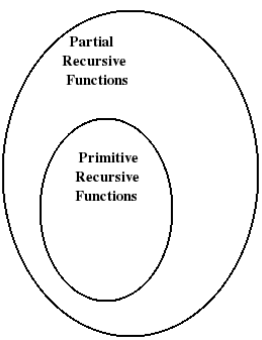Thus all primitive recursive functions are partial recursive functions.
Note that all partial recursive functions are computable functions.

Example 1:
Show that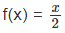is a partial recursive function over N.We may write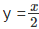Then,
x = 2y
2y − x = 0
Let
g(x, y) = |2y − x|
Let
g(x, y) = 0, for some x and y.
Let
f1(x) = μ[|2y − x| = 0]
Only for even values of x , f1(x) is defined. When x is odd, f1(x) is not defined. So f1 is partial recursive.
Since,= f1(x), f is a partial recursive function.

The document Recursive Function Theory Notes | Study Theory of Computation - Computer Science Engineering (CSE) is a part of the Computer Science Engineering (CSE) Course Theory of Computation.
All you need of Computer Science Engineering (CSE) at this link: Computer Science Engineering (CSE)

## Theory of Computation

18 videos|44 docs|39 tests
 Use Code STAYHOME200 and get INR 200 additional OFF

## Theory of Computation

18 videos|44 docs|39 tests

Track your progress, build streaks, highlight & save important lessons and more!

,

,

,

,

,

,

,

,

,

,

,

,

,

,

,

,

,

,

,

,

,

;